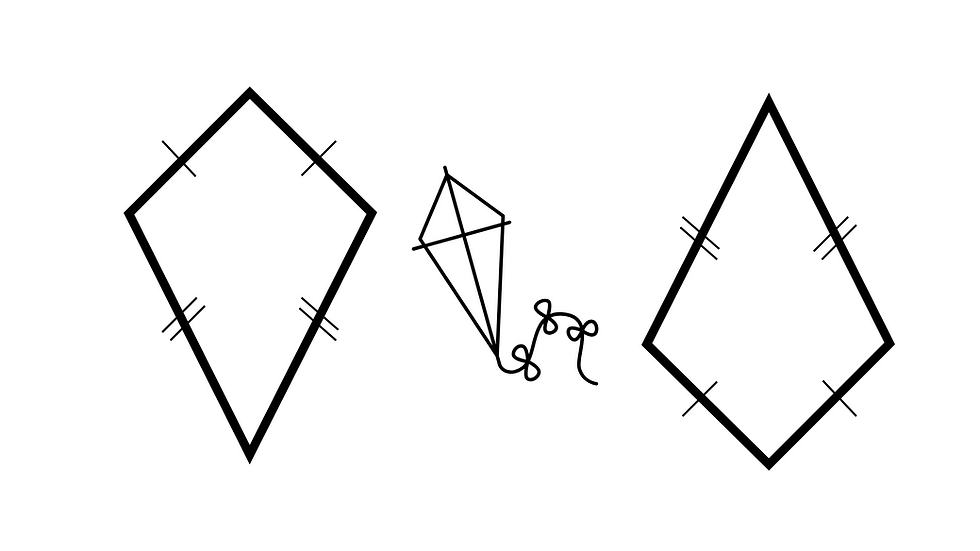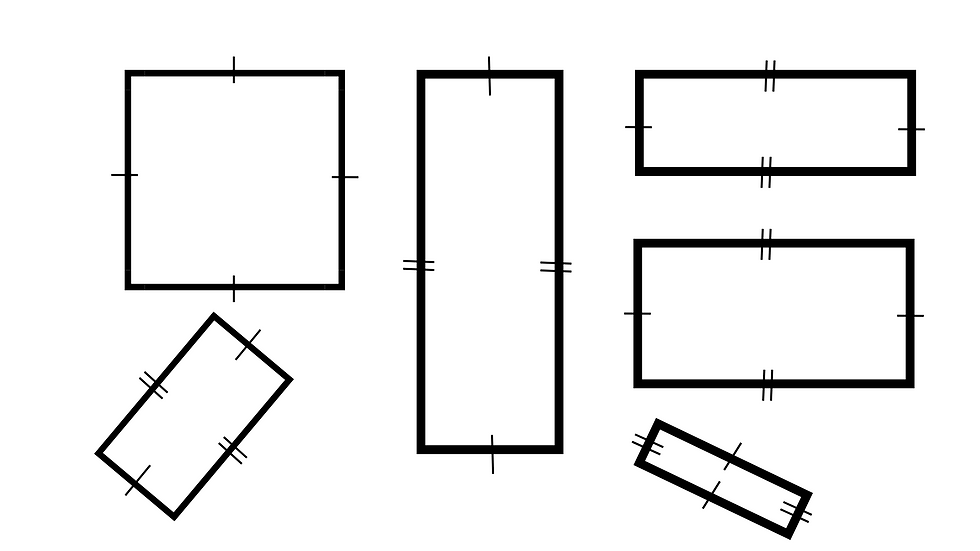top of page
Search

# How I wish I'd always taught... shape.A quadrilateral is a four-sided polygon in geometry. It can be defined as a shape with four straight sides that form four interior angles, which can be of any size. Some of the common types of quadrilaterals include squares, rectangles, parallelograms, rhombi, trapezoids, and kites. The sum of the interior angles of a quadrilateral is 360°.Kites

A kite is a quadrilateral with two pairs of equal length adjacent sides.Trapeziums

A trapezium (or trapezoid) is a type of quadrilateral in which only one pair of opposite sides are parallel. A trapezium has four sides, and the two non-parallel sides are called the legs of the trapezium. The parallel sides are called the bases of the trapezium, and the distance between them is called the height.Rhombi

A rhombus is a type of quadrilateral in which all sides are equal in length and opposite sides are parallel. It is sometimes referred to as a diamond shape.Rectangles

A rectangle is a type of quadrilateral in which all interior angles are right angles (90 degrees) and opposite sides are equal in length. It is characterized by having four straight sides and four right angles. A square is a special type of rectangle in which all sides are equal in length. The length and width of a rectangle determine its area, which can be calculated by multiplying the length by the width.Squares

A square is a type of quadrilateral in which all sides are equal in length and all interior angles are right angles (90 degrees). It is a special type of rectangle in which the length and width are equal. The sides of a square are also referred to as its edges. Each pair of opposite edges are parallel, and the diagonals of a square bisect each other at right angles and are equal in length. The area of a square can be calculated by squaring the length of one of its sides.

Symmetry

Symmetry (reflective symmetry) occurs when an object or patterns can be divided into equal parts. They create a mirror image. Rotational symmetry occurs when a a a shape. object or pattern can be rotated around a center point and it still looks the same. Transnational symmetry occurs when a shape, object or pattern can be moved a certain distance in a certain direction and still look the same.